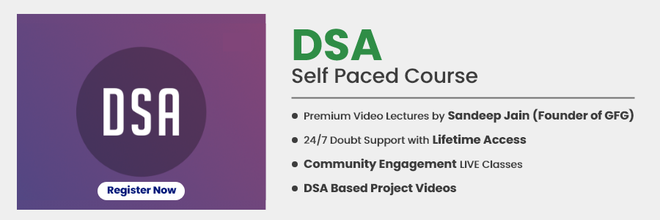Open in App
Not now

# Permutation and Combination

• Difficulty Level : Easy
• Last Updated : 07 Oct, 2022

## Permutation:

A Permutation is a collection or a combination of objects from a set where the order or the arrangement of the chosen objects does matter. In other words, a Permutation is an arrangement of objects in a definite order, For example, if we have two elements A and B, then there are two possible arrangements, ( A B ) and ( B A).

### Key Point

• nPn = n(n – 1) (n -2)… 3x2x1=n!
• nP0 = 1
• nP1 = n
• nPn-1 = n!
• nPr = n.n-1Pr-1 = n(n-1)n-2Pr-2

The number of permutations when ‘r‘ elements are arranged out of a total of ‘n’ elements is n Pr = n! / (n – r)! For example, let n = 4 (A, B, C and D) and r = 2 (All permutations of size 2). The answer is 4!/(4-2)! = 12. The twelve permutations are AB, AC, AD, BA, BC, BD, CA, CB, CD, DA, DB, and DC.

## Combination:

A Combination is the different selections of a given number of elements taken one by one, or some, or all at a time. For example, if we have two elements A and B, then there is only one way select two items, we select both of them.

### Key Point

• nCr​ is a natural number
• nC0​=(nCn​)=1
• nC1​=n
• nCr​=(nCn−r​)
• nCx​=nCy  ⇒x=y or x+y=n
• n.n−1Cr−1​=(n−r+1)×nCr−1​

The number of combinations when ‘r’ elements are selected out of a total of ‘n’ elements is n C r = n! / ((r !) x (n – r)!). For example, let n = 4 (A, B, C and D) and r = 2 (All combinations of size 2). The answer is 4!/((4-2)!*2!) = 6. The six combinations are AB, AC, AD, BC, BD, and CD.

Note: In the same example, we have different cases for permutation and combination. For permutation, AB and BA are two different things but for selection, AB and BA are the same.## Sample Problems

Question 1: How many words can be formed by using 3 letters from the word “DELHI”

Solution: The word “DELHI” has 5 different words. Therefore, required number of words = 5 P 3 = 5! / (5 – 3)!
Required number of words = 5! / 2! = 120 / 2 = 60

Question 2: How many words can be formed by using the letters from the word “DRIVER” such that all the vowels are always together?

Solution: In these types of questions, we assume all the vowels to be a single character, i.e., “IE” is a single character. So, now we have 5 characters in the word, namely, D, R, V, R, and IE. But, R occurs 2 times. => Number of possible arrangements = 5! / 2! = 60 Now, the two vowels can be arranged in 2! = 2 ways. => Total number of possible words such that the vowels are always together= 60 x 2 = 120

Question 3: In how many ways, can we select a team of 4 students from a given choice of 15?

Solution : Number of possible ways of selection = 15 C 4 = 15 ! / ((4 !) x (11 !))
Number of possible ways of selection = (15 x 14 x 13 x 12) / (4 x 3 x 2 x 1) = 1365

Question 4: In how many ways can a group of 5 members be formed by selecting 3 boys out of 6 boys and 2 girls out of 5 girls?

Solution : Number of ways 3 boys can be selected out of 6 = 6 C 3 = 6 ! / [(3 !) x (3 !)] = (6 x 5 x 4) / (3 x 2 x 1) = 20 Number of ways 2 girls can be selected out of 5 = 5 C 2 = 5 ! / [(2 !) x (3 !)] = (5 x 4) / (2 x 1) = 10 Therefore, total number of ways of forming the group = 20 x 10 = 200

Question 5: How many words can be formed by using the letters from the word “DRIVER” such that all the vowels are never together?

Solution: we assume all the vowels to be a single character, i.e., “IE” is a single character. So, now we have 5 characters in the word, namely, D, R, V, R, and IE. But, R occurs 2 times. => Number of possible arrangements = 5! / 2! = 60 Now, the two vowels can be arranged in 2! = 2 ways. => Total number of possible words such that the vowels are always together = 60 x 2 = 120 ,
total number of possible words = 6! / 2! = 720 / 2 = 360 Therefore, total number of possible words such that the vowels are never together 240

My Personal Notes arrow_drop_up